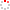CIOMP OpenIR  > 中科院长春光机所知识产出
 Calibration of polarized effect for time-divided polarization spectral measurement system Zhang, H.-Y.; Y. Li; C.-X. Yan and J.-Q. Zhang 2017 发表期刊 Guangxue Jingmi Gongcheng/Optics and Precision Engineering卷号 25期号:2 摘要 Aiming at the problems of polarizing effect in short wave infrared and measurement deviation in the polarization spectral measurement system built in lab, a system calibration model was established and a method to measure the correction coefficients of the model was proposed, thus the polarizing effect was calibrated precisely. Firstly, the main problem of the regular time-divided polarization spectral measurement system was described and the origin of the polarizing effect was analyzed. An ASD spectrometer which had a serious polarizing effect functioned as an analyzer according to the polarization transmission theory. Thereby a system calibration model was established and verified by comparing with the ideal model widely used. Then, a high precision fitting method of the correction coefficients was proposed for improving the measurement accuracy based on the analytical method. Experimental results indicate that the calibration precision is higher than 0.5%, which can fully satisfy the requirements of target polarization information processing in laboratory. This method has a great significance for the development and calibration of polarization spectra measurement system. 2017, Science Press. All right reserved. 收录类别 ei 语种 中文 文献类型 期刊论文 条目标识符 http://ir.ciomp.ac.cn/handle/181722/59429 专题 中科院长春光机所知识产出 推荐引用方式GB/T 7714 Zhang, H.-Y.,Y. Li,C.-X. Yan and J.-Q. Zhang. Calibration of polarized effect for time-divided polarization spectral measurement system[J]. Guangxue Jingmi Gongcheng/Optics and Precision Engineering,2017,25(2). APA Zhang, H.-Y.,Y. Li,&C.-X. Yan and J.-Q. Zhang.(2017).Calibration of polarized effect for time-divided polarization spectral measurement system.Guangxue Jingmi Gongcheng/Optics and Precision Engineering,25(2). MLA Zhang, H.-Y.,et al."Calibration of polarized effect for time-divided polarization spectral measurement system".Guangxue Jingmi Gongcheng/Optics and Precision Engineering 25.2(2017).
 条目包含的文件 下载所有文件 文件名称/大小 文献类型 版本类型 开放类型 使用许可 Calibration of polar（616KB） 期刊论文 作者接受稿 开放获取 CC BY-NC-SA 浏览 下载
 个性服务 推荐该条目 保存到收藏夹 查看访问统计 导出为Endnote文件 谷歌学术 谷歌学术中相似的文章 [Zhang, H.-Y.]的文章 [Y. Li]的文章 [C.-X. Yan and J.-Q. Zhang]的文章 百度学术 百度学术中相似的文章 [Zhang, H.-Y.]的文章 [Y. Li]的文章 [C.-X. Yan and J.-Q. Zhang]的文章 必应学术 必应学术中相似的文章 [Zhang, H.-Y.]的文章 [Y. Li]的文章 [C.-X. Yan and J.-Q. Zhang]的文章 相关权益政策 暂无数据 收藏/分享
 文件名： Calibration of polarized effect.pdf 格式： Adobe PDF